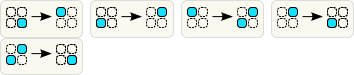Binary block cellular automata with Margolus neighborhood

A contrived title, but the thing is simple: consider an infinite field of square cells, where each cell can be dead: 0 or alive: 1 (thus binary). Now define the following evolution rule:

• Subdivide board into 2x2 cells. Each cell can be in one of 16 states.
• Transform every cell independently, using the same transformation function.
• Alternate subdivision by shifting the grid by (1,1).
This subdivision scheme is called Margolus neighborhood, and the whole system defines a cellular automaton.

Block cellular automata are interesting, because it is easy to make them reversible: just ensure that block transformation function is reversible (i.e. it is described by a transposition of 16 states). A well studied examples or rules are Critters, Billiard Ball machine, miscellaneous gas automata. My personal interest is the Single rotation rule.

Conserved quantities

Having done few experiments it is easy to notice that rules for such automata generally fall into 2 categories:

1. those that preserve population (BBM, Critters*, Single Rotation)
2. and those that don't (Tron and most random rules).
(*) in the first group, there is a sub-group of rules that invert population on each step (Critters is a good example), thus population restores every 2 steps. Such rules can be effectively simulated with 2 non-inverting rules applied alternatively.

Rules without population conservation almost inevitably exhibit chaotic growth for the most initial patterns. (A slightly paradoxical observation: for all reversible rules, there is no difference between evolution forward and backward in time, but a random initial pattern almost surely grows, like if there is a chosen direction of time).Small initial pattern in the rule without cell conservation. (Try live.)

The field tends to reach the state of maximal entropy, where roughly 50% of all cells are dead and 50% are alive.

Rules with conservation behave very different: they also strive to increase entropy, but this trend is limited by the conservation laws. Most interesting rules are in this class: Critters, BBM, Single Rotation.Rules with conservation (Single Rotation). Entropy growth is limited, complex emergent behavior appears. (Try live.)

Less trivial conserved quantities

So my question was: are there any rules that have some conserved quantity other than number of alive cells. And the answer is yes. There are at least 2 ways to construct such rules.

Weighted population

It is easy to notice that in the Margolus neighborhood scheme, sum cells of the board are always on the diagonal of the block, and others are on the anti-diagonal (black and white cells of a chessboard). We can assign different weights to white and black cells, and find a rule that preserves this modified weight.

There are 3 significantly different ways to assign positive weights to the "black" $$w_b$$ and "white" $$w_w$$ cells:

1. $$w_b = w_w = 1$$: both weights are the same, this is the usual population.
2. $$w_b = 1, w_w = 2$$: a non-trivial case.
3. $$w_b = 1, w_w > 2$$: equivalent to counting black and white cells separately, because 2 black cells in a block can never outweigh any white cell.
In rules that preserve the (1,3) weight, populations of cells on black and white cells of the board are not interacting, so the only non-trivial case here is (1,2) weighted sum.

The (1,2) weighted population of a block takes values in range [0, 6]. Block states are divided by it into 7 groups of equal weight:

Any rules that replaces block with another block of the same group, would preserve weighted population. Moreover, a rule that replaces block of weighted population $$w$$ with block of w.p. $$6-w$$, would be analog of the population inverting rule.

Some rules

The rules can be built by combining transpositions of states in the same group. It should be noted that most rules are anisotropic: they are not invariant to rotation bu 90°, patterns in such rules can't be rotated, only translated.

“Split and merge billiard&rdquo

This rule is defined by the following lookup table: [0,6,4,3,2,5,8,7,1,9,10,11,12,13,14,15], itis illustrated by the diagram below.Substitution diagram for the split and merge billiard rule. Other block states remain unchanged.

It is an analog of the “Billiard Ball Machine” rule, but two anti-diagonal balls may merge on collision and create one diagonal.

Rule [15,14,13,10,11,3,9,1,7,8,12,2,5,4,6,0]

This rule inverts weighted population on each step, thus after 2 generations weighted population is restored. This rule is relatively rare example of its family, because it have spaceships, moving in both directions along both diagonals (of course, these are different spaceships). Several tens minutes of random search discovered 35 different moving patterns.

Other rules with spaceships

There is plenty of rules, supporting spaceships. Some of them are:

Negative weights

It is also possible to assign negative weights to the block cells. In rules preserving resulting invariant, total number of alive cells can grow infinitely, while invariant remains constant. This usually makes such rules “explosive&rdquo: most initial patterns grow ore or less chaotically.

An easy way to produce such rules is to take a rules with positive weights and consider it acting on a board, where half of cells is alive, forming a chessboard pattern. Then build a rule, describing evolution of the XOR between some field and the infinite chess pattern. For example, for "single rotation" resulting rule is [0,13,2,3,4,5,6,7,11,9,10,1,12,8,14,15]. It is partially "explosive", but supports some simple spaceships, moving along main diagonal.Zaratustra said…
"a random initial pattern almost surely grows"

Seems to me that'd be intuitive, if the average end-goal is roughly 50% of cells filled up.

What about initial patterns with a population above 50% of the grid? One would expect them to almost surely shrink.